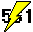#I'm currently working on a new algorithm which seems very efficient for detecting composite numbers (including many pseudoprimes, strong pseudoprimes and Carmichael numbers). For the most part, the algorithm can also give one factor or more which can be very large (up to thousands of digits).
This algorithm (which doesn't use Fermat test, nor P+/-1 test, nor elliptic curve methods) is mostly based on personal conjectures, so I can't give it to you because it is not yet correctly studied and have more possibilities. Nevertheless, I give you here a special version of this program, limited to the factorization of the numbers which passes the Fermat test in base 3 (for example : some pseudoprimes, some strong pseudoprimes, and all the Carmichael).
The most interesting thing is the speed of the program compared to existing algorithms. Indeed, the algorithm is in polynomial time (e.i.: it takes a time proportional to log(N)). Here are some execution times on my AMD-Thunderbird 900Mhz computer :

 Number of digits of the number Average factorization time (one test) 300 1 sec. 600 8 sec. 1200 52 sec. 2400 320 sec.

And here are some output examples :

```MultiFact V1.0r    R.Lifchitz    Sun Mar 25 19:23:57 2001 ```

```Number = 68528663395046912244223605902738356719751082784386681071 (186 bits) Factor = 18215745452589259639 (64 bits)```

``` Secondary certificate : 6957048689075372916369533```

```-------------------------------------------------------------------------------- MultiFact V1.0r    R.Lifchitz    Sun Mar 25 19:25:09 2001 ```

```Number = 21253673841183908899878595198761054000000000000000000000000000000000000000000000000000000000000000000000000000000000000000000000000000000000000000000000000000000000000000000000000000000000000000000000000000000000000000000000000000000000000000000000000000000000000000000000000000000000000000000000000000000000000000000000000000000000000000000000000000000000000000000000000000000000000000000000000000000000000000000000000000000000000000000000000000000000000000000000000000000000000000000000000000000000000000000000000000000000000000000000000000000000000000000000000000000000000000000000000000000000000000000000000000000000000000000000000000000000000000000000000000000000000000000000000000000000000000000000000000000000000000000000000000000000000000000000000000000000000000000000000000000000000000000000000000000000000000000000000000000000000000000000000000000000000000000000000000000000000000000000000000000000000000000000000000000000000000000000000000000000000000000000000000000000000000000000000000000000000000000000000000002556119730575676979423710000000000000000000000000000000000000000000000000000000000000000000000000000000000000000000000000000000000000000000000000000000000000000000000000000000000000000000000000000000000000000000000000000000000000000000000000000000000000000000000000000000000000000000000000000000000000000000000000000000000000000000000000000000000000000000000000000000000000000000000000000000000000000000000000000000000000000000000000000000000000000000000000000000000000000000000000000000000000000000000000000000000000000000000000000000000000000000000000000000000000000000000000000000000000000000000000000000000000000000000000000000000000000000000000000000000000000000000000000000000000000000000000000000000000000000000000000000000000000000000000000000000000000000000000000000000000000000000000000000000000000000000000000000000000000000000000000000000000000000000000000000000000000000000000000000000000000000000000000000000000000000000000000000000000000000000000000000000000000000000000000000000000000000000000000000000000000091463013041400000000000000000000000000000000000000000000000000000000000000000000000000000000000000000000000000000000000000000000000000000000000000000000000000000000000000000000000000000000000000000000000000000000000000000000000000000000000000000000000000000000000000000000000000000000000000000000000000000000000000000000000000000000000000000000000000000000000000000000000000000000000000000000000000000000000000000000000000000000000000000000000000000000000000000000000000000000000000000000000000000000000000000000000000000000000000000000000000000000000000000000000000000000000000000000000000000000000000000000000000000000000000000000000000000000000000000000000000000000000000000000000000000000000000000000000000000000000000000000000000000000000000000000000000000000000000000000000000000000000000000000000000000000000000000000000000000000000000000000000000000000000000000000000000000000000000000000000000000000000000000000000000000000000000000000000000000000000000000000000000000000000000000000000000000000000000000000000000001 (10210 bits) Factor = 45731506520700000000000000000000000000000000000000000000000000000000000000000000000000000000000000000000000000000000000000000000000000000000000000000000000000000000000000000000000000000000000000000000000000000000000000000000000000000000000000000000000000000000000000000000000000000000000000000000000000000000000000000000000000000000000000000000000000000000000000000000000000000000000000000000000000000000000000000000000000000000000000000000000000000000000000000000000000000000000000000000000000000000000000000000000000000000000000000000000000000000000000000000000000000000000000000000000000000000000000000000000000000000000000000000000000000000000000000000000000000000000000000000000000000000000000000000000000000000000000000000000000000000000000000000000000000000000000000000000000000000000000000000000000000000000000000000000000000000000000000000000000000000000000000000000000000000000000000000000000000000000000000000000000000000000000000000000000000000000000000000000000000000000000000000000000000000000000000000000000001 (3404 bits)```

``` Secondary certificate : 7334761488390314031395411```

```-------------------------------------------------------------------------------- MultiFact V1.0r    R.Lifchitz    Tue Mar 27 20:52:10 2001```

```Number = 12831493933205463446565634841283200779383250905791025414412317860607969755447225326496549183066207149493847677427330635581154060218847953981172822981696394671076724138434769129651403024830325564451621955270750534389398898562336989370932486325759454411581589772882894855240149165066140627411590302024368652372154076383514898206358769169546291476955030264556103951159258507905191427701736145390300780838719352497935219030150738725732343625618973826793281510143247790864513680829504865706487237447547029241799996156235316636030135821960972078898166375012508164447941721856148684483962733573385949221332618389292520091602459306734162659882713169463546905576388191275247392748082132308318086619157171025936480226415120544712245898391373246545554793953125039475038450967110070443784393623147874856456383779052847462264625825660084154868991767819772572287782651310679745785765772622538195747802861755718010811119988241874381678570395242410285915534984772237507397502023943838865155720957359975000746244049134034884210570649724927734948841827903076167740702401295545567968264379356960611037348114217687189771587848680685656456513248660235597186628740652688257008790667959761825007171051404716592500394635598795927893084998842226600761924352604785296547408289630977188968508847095452306439812283762726345505972640573209027939898014678098693651713929694742749839553865062545314190587376445590433997986674833891897254542149735546288081635529237109861598503160926605668930491827275355234989187290060145606998175153612697404266670371695150178379406990149434200618718404349341017794183058061077566435573210158603351038163648268794928681948070150447903505183260095248508456091334522526246565997542877211892480647871913679688414706657614473834991015884325385502757704030753475423342282369512790905592308878355930952213992251745513878429571984667060489332830146393054827994246342167421629707268134062762839443048862681376602137050777385423428199591719489676325794735592557921232448016304423886818590755568747660225186722208169237286008000566589197658143531791903140234407345526812075489842853240020350851198470470928440194715179138873816702704233987503577553871216622417366846316690343256185146268307924342734062829592763219610741234763007820328480328910325187297655685663018073001931148433581918966023204679078995310054194506810807747560524179111848328541678848412885199599061133039978144640273134736868518114094324478023479700817986945109266688923397935120312613436697147893092863788121895360025745323583726396403555823273130236811674631889822440819469347789344904217116654229072319100308040496717392084509591656810583203066533358715538957413779959414546414806392493815730418732908422549307238770833595768336621424357958604081352132210014796448635403581110254752387370542328656935184272923733956605374867565037391915033089357588049994224504294050295041798036149203758015022579106756072527749625433583467276202023042519271013638526704420181086068823259947596719871068928687809398990386828303423113854254792001870716156623701195059421972267785288026551501670216189580040655547521765303450871650973931914026840668725914946600879064198084110579671208467755047215092111898510130376564053919851275496485385178502431197756789857872526479101496034073031296829766130083888317839589246331705178126870311203771676825438078813012636026217957010805972496416449244632790897118920812640709554170195625171989028404641571830453284880122583315587253702198389747840550531050647016515923209485853783485582040983981823606821447704669378748339365006425468919564348017762551040211408370227725253944012566319726245085755500311569944334405662158361300598663915726534054884400732567326819363190916680382691237560869308052775074983146943054150266519553 (12322 bits) Factor = 10206925758549975668412716780406450208681372010347250541586701015597947712956123109676084885488942263469703697352415567037836390301582626354141657754011276405840348855972940276077664169551430506447210707060590260847714033973680236126684952474460231174500190078107967701841118897837137470411510141405497622272141131255192242470603509423629253398941993175828788962166474835999345623623081689141153602419958651464824797724924464301561212064951843613767665949545407099162814195993003048988421415752092252681961119141823495174343506817524359786308731452893338730042762097534372449338555984073383905257696563365782160361332777324545150057386441244014272955481605733577353880371389483305368742208659280130078307445905864159257798354501049904259230033832153971444428323459104911403437626290164394243182160000086860341824581334427218570610723272407107094473125162406890567615947833826621856664451798065006201238104911734609823216666963576193841551036420038474814897277842073973352513823534338316699445294027201872790145260138044319423573732736620077888414541075335638234934765342750813245798680183576995056022183898570501328765123243923593389200282957313116973430175696768941169375408776725123507539236991686386361211284027475508132838593067420256543082107737634680549540087894129283576081757902095558646255738582210878691601571807980734593626203663074520670324105330825878429881765814496919375199117794564917149975697711280600339671141580702536930422163544264762268922693273974334401575359967076898159569658550772034706249074800724882347881743701353133176962985180837863592815345504504853504551852586939013349987070255101572088140153715730682745270408220089984872861100089182663806707422837923219072115185936480597684172395770880210702042520438976119179986437737819730561338521449865751981327250980682787159809584278362595988479017967266719835869055961438093313 (6153 bits)```

``` Secondary certificate : 8332583672750517695962507```

```-------------------------------------------------------------------------------- ```

``` ```The last example is quite impressive since a factor of more than 1800 digits is found in about 19 minutes !

How to use the program :

Enter your PSP-3 number on one line in a file called "number.fic", in the directory of the program. The program will open it, will trial-factor it, will effectively verify that it is really a PSP-3 number, will start a sequence of 10 consecutive factoring tests, and will stop as soon as a factor is found. The computation time for each test is written on the screen and all the found factors are written in a file called "results.fic". The factor is a certificate of the compositeness, but a secondary certificate is also written : it contains various parameters used for the computation.

Have fun !

Click here to download the program "MultiFact v1.1r" (Win9x version, zip archive : 58 Ko) :Version 1.1r : Improves factorization rate